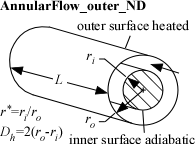Contents - Index

AnnularFlow_outer_NDProcedure AnnularFlow_outer_ND(Re, Pr, LoverD_h, r|star, relRough: Nusselt_T,Nusselt_H, f) returns the lower and upper bounds on the Nusselt number for developing or fully-developed flow in an annulus characterized by inner and outer radii.  The inner wall of the annulus is assumed to be insulated so heat transfer occurs across the outer wall.  The area used for heat transfer should be the area of this outer wall.  The procedure also returns the friction factor for these conditions.

Inputs:

Re - Reynolds number based on properties evaluated at the bulk average temperature

Pr - Prandtl number of the fluid evaluated at the bulk average temperature

LoverD_h - ratio of tube length to hydraulic diameter.

r|star - ratio of the inner to the outer radius of the annulus   This value should be <=1 and >0

RelRough - the ratio of the dispersions on the wall of the tube to the tube diameter (must be between 0 and 0.05)

Outputs:

Nusselt_T - lower bound on the Nusselt number assuming a constant outer annular wall temperature

Nusselt_H - upper bound on the Nusselt number assuming a constant outer annular wall heat flux

f -  friction factor [-]

Notes:

This procedure is called by AnnularFlow_outer. It calls AnnularFlow_Laminar and PipeFlow_Turbulent to obtain the appropriate Nusselt numbers and the friction factor.

The procedure will determine if the flow is laminar or turbulent.  Transitional flow is assumed to occur for Reynolds numbers (based on hydraulic diameter) between 2300 and 3000 and interpolation is applied between the laminar and turbulent correlations.

The parameter LoverD_h is used to apply a developing flow correction for the turbulent flow, if necessary.  Set LoverD_h to a large number if developing flow corrections are not applicable.

Two values of the Nusselt number coefficient are returned that should provide upper and lower bounds.  These coefficients are both for the outer annular wall with an insulated inner wall.  They both correspond to fully developed flow. For turbulent flow, these values are identical.

Example:

Re=1500

Pr=0.72

LoverD=20

r|star=0.5

relRough=0

call AnnularFlow_outer_ND(Re, Pr, LoverD, r|star, relRough: Nusselt_T,Nusselt_H, f)

{Solution:

f=0.1008 [-]

Nusselt_H=7.608

Nusselt_T=6.882 [-]}